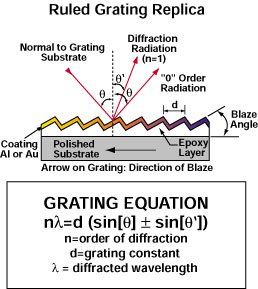# What is the grating equation?

The grating equation is an expression that can be used to calculate the diffraction angle from light incident to the grating's grooved surface for a particular order, given the wavelength and angle of incidence for that light. By knowing where the light will be diffracted, the grating can be rotated to redirect the light to a desired location. The grating equation and accompanying illustration is shown below: